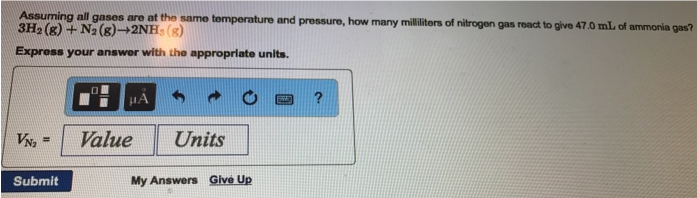# Problem: Assuming all gases are at the same temperature and pressure, how many milliliters of nitrogen gas react to give 47.0 mL of ammonia gas? 3H2(g) + N2(g) → 2NH3 (g). Express your answer with the appropriate units.

###### FREE Expert SolutionAssuming all gases are at the same temperature and pressure, how many milliliters of nitrogen gas react to give 47.0 mL of ammonia gas? 3H2(g) + N2(g) → 2NH3 (g). Express your answer with the appropriate units.